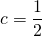# Solution assignment 09 Root equations

### Assignment 9

For which value(s) of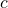is the line: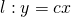tangent to the graph of: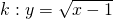### Solution

First we try to find possible intersection points ofand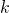. Next we try to choosesuch that the intersection points coincide (or graphs have 'one' intersection point).
We find these intersection points ofandfrom: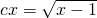After squaring we find: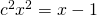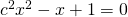The solutions of this quadratic equations indicate the intersection points ofand. The discriminant determines whether there are intersection points and if so, how many: 0, 1 or 2. We want that the graphs ofandare tangent and thus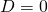: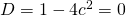so: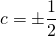The value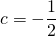does not satisfy because a square root cannot take a negative value. Therefore the solution is: